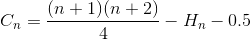# Insertion Sort

suggest change

## Remarks

When we analyze the performance of the sorting algorithm, we interest primarily on the number of comparison and exchange.

## Average Exchange

Let En be the total average number of exchange to sort array of N element. E1 = 0 (we do not need any exchange for array with one element). The average number of exchange to sort N element array is the sum of average number of number of exchange to sort N-1 element array with the average exchange to insert the last element into N-1 element array.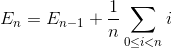Simplify the summation (arithmetic series)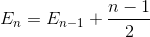Expands the termSimplify the summation again (arithmetic series)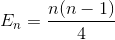## Average Comparison

Let Cn be the total average number of comparison to sort array of N element. C1 = 0 (we do not need any comparison on one element array). The average number of comparison to sort N element array is the sum of average number of number of comparison to sort N-1 element array with the average comparison to insert the last element into N-1 element array. If last element is largest element, we need only one comparison, if the last element is the second smallest element, we need N-1 comparison. However, if last element is the smallest element, we do not need N comparison. We still only need N-1 comparison. That is why we remove 1/N in below equation.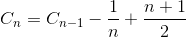Simplify the summation (arithmetic series)Expand the term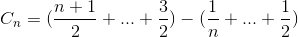Simplify the summation again (arithmetic series and harmonic number)